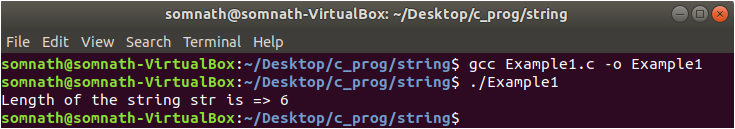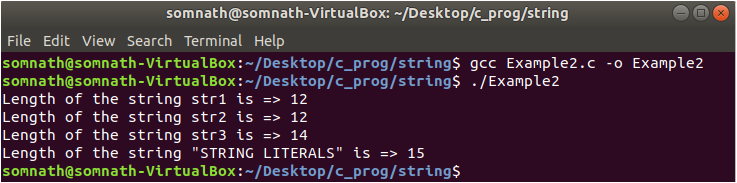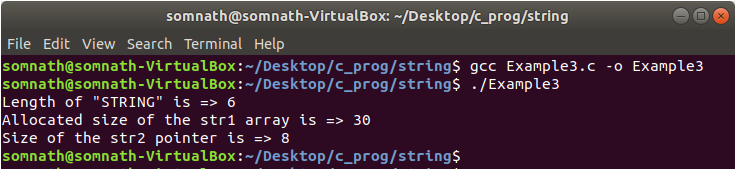16 C
Jaipur
Friday, January 22, 2021

# String Length in C Language – Linux Hint

### JioMeet video calling platform crosses 15 millions users

A string in C language is an array of characters that is terminated with a null character (). The string length is the number of characters in a string. In the string length ‘,’ a character is not counted.

In the example shown above, the length of the string str is 6.

In this tutorial, first, we will show how to use a user defined function to calculate length of a string, and then we will show you a built-in library function strlen(). We also show you the uses of the sizeof operator for string literals.

### String Length Using User Defined Function

You can write a user defined function which returns the number of characters in a string.

//Example1.c
#include<stdio.h>

int stringLength(char *str)
{
int i=0;

while(str[i] != ) i++;

return i;
}

int main()
{
char str= “STRING”;

printf(“Length of the string str is => %dn,stringLength(str));

return 0;
}Here, we iterate the while loop from i = 0 until we do not encounter the ‘’ character. The value of i is increased by 1 in each iteration of the while loop. When the loop ends, the value of i is the length of the string.

### String Length Using Built-In Library Function

The built-in library function strlen() can also be used to determine string length.

strlen() function:

Syntax:

size_t strlen (const char *str)

Argument: This function takes an argument of the type pointer to char.

Return value: This function returns the length of the string str. Note that size_t is just an alias of an unsigned integer.

//Example2.c
#include<stdio.h>
#include<string.h>

int main()
{
char str1=“STRING ARRAY”;
char *str2;
char *str3;

str2 = str1;
str3 = “STRING POINTER”;

printf(“Length of the string str1 is => %ldn,strlen(str1));
printf(“Length of the string str2 is => %ldn,strlen(str2));
printf(“Length of the string str3 is => %ldn,strlen(str3));
printf(“Length of the string “STRING LITERALS” is => %ldn,strlen(“STRING LITERALS”));

return 0;
}Here, we pass string array, string pointer, and string literal to the strlen function, and the function returns the length of the string.

### String Length Using sizeof Operator

We also can use the sizeof operator for string length (only for string literal). But, we have to subtract 1 from the value returned by this operator, because it also counts the’’ character. For array and pointer, the sizeof operator returns the allocated size of the array and the pointer, respectively.

//Example3.c
#include<stdio.h>

int main()
{
char str1 = “STRING”;
char *str2 =str1;

printf(“Length of “STRING” is => %dn,(sizeof(“STRING”) 1));
printf(“Allocated size of the str1 array is => %dn,sizeof(str1));
printf(“Size of the str2 pointer is => %dn,sizeof(str2));

return 0;
}Here, in Line no 9, we pass the string literal “STRING” and get the size, including the ‘’ character. So, we subtract 1 and get the actual size of the string.

When we pass an array to the sizeof operator, it returns the allocated size of the array, which is 30, and when passing a character pointer, it returns the size of the pointer.

### Conclusion

So, in this tutorial, we have shown you how string length can be calculated in various ways. You can use in your code whichever method is best suited for you.